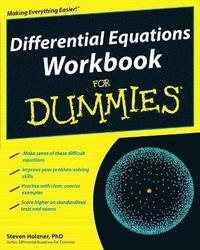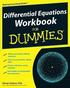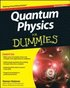Fler böcker inom
Format
Språk
Engelska
Antal sidor
320
Utgivningsdatum
2009-07-17
Upplaga
1
Förlag
John Wiley & Sons Inc
Illustrationer
black & white illustrations, black & white tables
Dimensioner
260 x 203 x 19 mm
Vikt
444 g
Antal komponenter
1
ISBN
9780470472019

### Du kanske gillar# Differential Equations Workbook For Dummies

181
Skickas inom 5-8 vardagar.
Fri frakt inom Sverige för privatpersoner.
Finns även som
169
Tips, tricks and lots of practice to help students get a handle on these complex calculus problems Pre-calculus classes prepare students for studies in calculus and other advanced Differential equations are essential in physics, economics, engineering, and many other scientific and technical disciplines. This new Dummies Workbook is a hands-on companion for students grappling with differential equations in classes such as calculus, AP calculus, physics, and engineering. It features numerous practice exercises and clear, concise examples to help students improve their problem-solving skills and score better in science, math, and standardized tests. This practice-oriented workbook covers such challenging topics as first-order and second-order differential equations, constant coefficients, undetermined coefficients, variation of parameters, initial-value problems, the Laplace transform, and more.

## Passar bra ihop

1.2. +
3.De som köpt den här boken har ofta också köpt Quantum Physics For Dummies av Steven Holzner (häftad).

## Kundrecensioner

Har du läst boken? Sätt ditt betyg »

## Övrig information

Steven Holzner, PhD, served on the faculty of Cornell University and Massachusetts Institute of Technology.? He is an award-winning author who has written Physics For Dummies, Quantum Physics For Dummies, and more.

## Innehållsförteckning

Introduction. Part I: Tackling First Order Differential Equations. Chapter 1: Looking Closely at Linear First Order Differential Equations. Chapter 2: Surveying Separable First Order Differential Equations. Chapter 3: Examining Exact First Order Differential Equations. Part II: Finding Solutions to Second and Higher Order Differential Equations. Chapter 4: Working with Linear Second Order Differential Equations. Chapter 5: Tackling Nonhomogeneous Linear Second Order Differential Equations. Chapter 6: Handling Homogeneous Linear Higher Order Differential Equations. Chapter 7: Taking On Nonhomogeneous Linear Higher Order Differential Equations. Part III: The Power Stuff: Advanced Techniques. Chapter 8: Using Power Series to Solve Ordinary Differential Equations. Chapter 9: Solving Differential Equations with Series Solutions Near Singular Points. Chapter 10: Using Laplace Transforms to Solve Differential Equations. Chapter 11: Solving Systems of Linear First Order Differential Equations. Part IV: The Part of Tens. Chapter 12: Ten Common Ways of Solving Differential Equations. Chapter 13: Ten Real-World Applications of Differential Equations. Index.